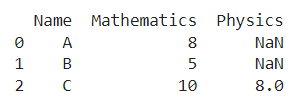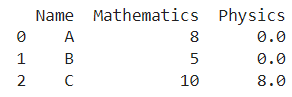# How To Replace NaN Values By Zeroes In A Column Of A Pandas DataFrame

A DataFrame is the primary data structure of the Pandas library in Python and is commonly used for storing and working with tabular data.

A common operation that could be performed on such data is to replace nan values with zeroes in a column in order to make it easier to handle.

To start working with Pandas, we first need to import it:

``import pandas as pd``

We’ll also need the NumPy library for this to specify NaN values. To work with NumPy, we first need to import it in the following manner:

### Python 3 Code

``import numpy as np``

## Running Example

Let us understand this operation with the help of an example. Consider the following DataFrame containing 3 students with names A, B, and C and their corresponding marks (out of 10) for two subjects, Mathematics and Physics.Code snippet for generating the above DataFrame :

### Python 3 Code :

``````import pandas as pd
import numpy as np

# Dictionary for our data
data = {'Name' : ['A', 'B', 'C'], 'Mathematics' : [8, 5, 10], 'Physics' : [np.nan, np.nan, 8]}

# DataFrame for the dictionary
df = pd.DataFrame(data=data)

# Printing the DataFrame
print(df)``````

Here, data is a dictionary we created to initialize the DataFrame.

For this, we use the .DataFrame() function of the Pandas library which takes the dictionary as an argument and returns the required DataFrame. One important thing to note here is that the first two rows of the column physics have NaN values.

Now, let’s say we need to replace nan values in a column with zeroes. Here the column of interest is physics. The resulting output would look like this :Let us look at different ways of performing this operation on a given DataFrame :

## Replace NaN with Zero by using the Series.fillna() function

In this method, we use the Series.fillna() function to replace nan values with zeroes in a column of the DataFrame.

This function returns the updated Series of the column and the changes are not made in place by default therefore reassignment is required.

Let us take a look at the corresponding code snippet and generated output for this method:

``````# Importing required libraries
import pandas as pd
import numpy as np

# Dictionary for our data
data = {'Name' : ['A', 'B', 'C'], 'Mathematics' : [8, 5, 10], 'Physics' : [np.nan, np.nan, 8]}

# DataFrame for the dictionary
df = pd.DataFrame(data=data)

# Performing the operation
df['Physics'] = df['Physics'].fillna(0)

# Printing the result
print(df)``````

Output :Instead of using df[‘Physics’] to access the physics column, we could also simply use the dot operator in the form of df.Physics to get the same result.

Let us take a look at the corresponding code snippet and generated output for this method:

``````# Importing required libraries
import pandas as pd
import numpy as np

# Dictionary for our data
data = {'Name' : ['A', 'B', 'C'], 'Mathematics' : [8, 5, 10], 'Physics' : [np.nan, np.nan, 8]}

# DataFrame for the dictionary
df = pd.DataFrame(data=data)

# Performing the operation
df.Physics = df.Physics.fillna(0)

# Printing the result
print(df)``````

Output :In case we want to perform the above operation in an in place manner, we pass the parameter inplace as True inside the replace() function.

Here, we do not require reassignment. Let us take a look at the corresponding code snippet and generated output for this method:

``````# Importing required libraries
import pandas as pd
import numpy as np

# Dictionary for our data
data = {'Name' : ['A', 'B', 'C'], 'Mathematics' : [8, 5, 10], 'Physics' : [np.nan, np.nan, 8]}

# DataFrame for the dictionary
df = pd.DataFrame(data=data)

# Performing the operation
df.Physics.fillna(0, inplace = True)

# Printing the result
print(df)``````

Output :## Replace NaN with Zero by using the Series.replace() function

In this method, we use the Series.replace() function to replace nan values with zeroes in a column of the DataFrame.

The first parameter is the element that needs to be replaced, here np.nan.

The second parameter is the element by which it has to be replaced, here 0.

We pass the regex parameter as True so that the function considers it as a regular expression, also known as regex.

This function returns the updated Series of the column. The changes are not made in place by default so reassignment is required.

Let us take a look at the corresponding code snippet and generated output for this method :

``````# Importing required libraries
import pandas as pd
import numpy as np

# Dictionary for our data
data = {'Name' : ['A', 'B', 'C'], 'Mathematics' : [8, 5, 10], 'Physics' : [np.nan, np.nan, 8]}

# DataFrame for the dictionary
df = pd.DataFrame(data=data)

# Performing the operation
df['Physics'] = df['Physics'].replace(np.nan, 0, regex = True)

# Printing the result
print(df)``````

Output :In case, we want to perform the above operation in an in place manner, we pass the parameter inplace as True inside the replace() function.

Here, we do not require reassignment. Let us take a look at the corresponding code snippet and generated output for this method:

``````# Importing required libraries
import pandas as pd
import numpy as np

# Dictionary for our data
data = {'Name' : ['A', 'B', 'C'], 'Mathematics' : [8, 5, 10], 'Physics' : [np.nan, np.nan, 8]}

# DataFrame for the dictionary
df = pd.DataFrame(data=data)

# Performing the operation
df['Physics'].replace(np.nan, 0, regex = True, inplace = True)

# Printing the result
print(df)``````

Output :## Conclusion

In this topic, we have learned to replace nan values with zeroes in a column of an existing Pandas DataFrame, following a running example of test scores of students in different subjects, thus giving us an intuition of how this concept could be applied in real-world situations. Feel free to reach out to info.javaexercise@gmail.com in case of any suggestions.

Trending